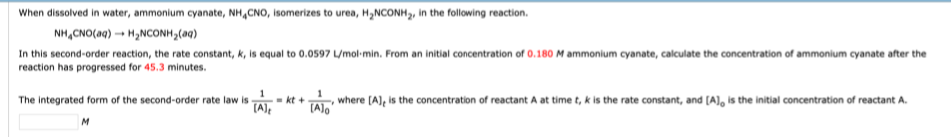# When dissolved in water, ammonium cyanate, NH4CNO, isomerizes to urea, H2NCONH2, in the following reaction. NH4CNO(aq) H2NCONH2(aq) In this second-order reaction, the rate constant, k, is equal to 0.0597 L/mol•min. From an initial concentration of 0.180 M ammonium cyanate, calculate the concentration of ammonium cyanate after the reaction has progressed for 45.3 minutes. The integrated form of the second-order rate law is where [A]t is the concentration of reactant A at time t, k is the rate constant, and [A]o is the initial concentration of reactant A.